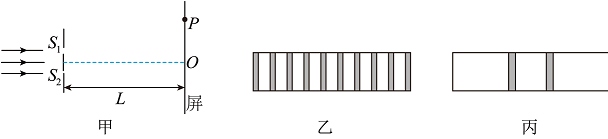$\text{A.}$ 图乙是光的双缝干涉图样，当光通过狭缝时，也发生了衍射 $\text{B.}$ 遮住一条狭缝，另一狭缝宽度增大，其他条件不变，图丙中亮条纹宽度增大 $\text{C.}$ 照射两条狭缝时，增加L，其他条件不变，图乙中相邻暗条纹的中心间距增大 $\text{D.}$ 照射两条狭缝时，若光从狭缝 S1、S2 到屏上P点的路程差为半波长的奇数倍，P点处一定是暗条纹

ACD

#### 解析：

A. 由图可知, 图乙中间部分等间距条纹, 所以图乙是光的双缝干涉图样, 当光通过狭缝时, 同时也发生衍 射, 故 A 正确;

B. 狭缝越小, 衍射范围越大, 衍射条纹越宽, 遮住一条狭缝, 另一狭缝宽度增大, 则衍射现象减弱, 图丙 中亮条纹宽度减小, 故 B 错误;

C. 根据条纹间距公式 $\Delta x=\frac{L}{d} \lambda$ 可知照射两条狭缝时, 增加 $L$, 其他条件不变, 图乙中相邻暗条纹的中心 间距增大，故 C 正确;

D. 照射两条狭缝时, 若光从狭缝 $S_1 、 S_2$ 到屏上 $P$ 点的路程差为半波长的奇数倍, $P$ 点处一定是暗条纹, 故 D 正确。